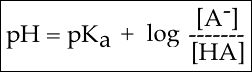# pKa#

## pKa

pKa is defined as the negative log10 of the dissociation constant of an acid, its Ka. Therefore, the pKa is a quantitative measure of how easily or how readily the acid gives up its proton [H+] in solution and thus a measure of the "strength" of the acid. Strong acids have a small pKa, weak acids have a larger pKa.

The most common acid we will talk about in BIS2A is the carboxylic acid functional group. These acids are typically weak acids, meaning that they only partially dissociate (into H+ cations and RCOO- anions) in neutral solution. HCL (hydrogen chloride) is a common strong acid, meaning that it will fully dissociate into H+ and Cl-.

Note that the key difference in the figure below between a strong acid or base and a weak acid or base is the single arrow (strong) versus a double arrow (weak). In the case of the single arrow you can interpret that by imagining that nearly all reactants have been converted into products. Moreover, it is difficult for the reaction to reverse backwards to a state where the protons are again associated with the molecule there were associated with before. In the case of a weak acid or base, the double-sided arrow can be interpreted by picturing a reaction in which:

1. both forms of the conjugate acid or base (that is what we call the molecule that "holds" the proton - i.e. CH3OOH and CH3OO-, respectively in the figure) are present at the same time and
2. the ratio of those two quantities can change easily by moving the reaction in either direction.Figure 1. An example of strong acids and strong bases in their protonation and deprotonation states. The value of their pKa is shown on the left. Attribution: Marc T. Facciotti

Electronegativity plays a role in the strength of an acid. If we consider the hydroxyl group as an example, the greater electronegativity of the atom or atoms (indicated R) attached to the hydroxyl group in the acid R-O-H results in a weaker H-O bond, which is thus more readily ionized. This means that the pull on the electrons away from the hydrogen atom gets greater when the oxygen atom attached to the hydrogen atom is also attached to another electronegative atom. An example of this is HOCL. The electronegative Cl polarizes the H-O bond, weakening it and facilitating the ionization of the hydrogen. If we compare this to a weak acid where the oxygen is bound to a carbon atom (as in carboxylic acids) the oxygen is bound to the hydrogen and carbon atom. In this case, the oxygen is not bound to another electronegative atom. Thus the H-O bond is not further destabilized and the acid is considered a weak acid (it does not give up the proton as easily as a strong acid).Figure 2. The strength of the acid can be determined by the electronegativity of the atom the oxygen is bound to. For example, the weak acid Acetic Acid, the oxygen is bound to carbon, an atom with low electronegativity. In the strong acid, Hypochlorous acid, the oxygen atom is bound to an even more electronegative Chloride atom.

In Bis2A you are going to be asked to relate pH and pKa to each other when discussing the protonation state of an acid or base, for example, in amino acids. How can we use the information given in this module to answer the question: Will the functional groups on the amino acid Glutamate be protonated or deprotonated at a pH of 2, at a pH of 8, at a pH of 11?

In order to start answering this question we need to create a relationship between pH and pKa. The relationship between pKa and pH is mathematically represented by Henderson-Hasselbach equation shown below, where [A-] represents the deprotonated form of the acid and [HA] represents the protonated form of the acid.Figure 3. The Henderson-Hasselbach equation

A solution to this equation is obtained by setting pH = pKa. In this case, log([A-] / [HA]) = 0, and [A-] / [HA] = 1. This means that when the pH is equal to the pKa there are equal amounts of protonated and deprotonated forms of the acid. For example, if the pKa of the acid is 4.75, at a pH of 4.75 that acid will exist as 50% protonated and 50% deprotonated. This also means that as the pH rises, more of the acid will be converted into the deprotonated state and at some point the pH will be so high that the majority of the acid will exist in the deprotonated state.Figure 4. This graph depicts the protonation state of acetic acid as the pH changes. At a pH below the pKa, the acid is protonated. At a pH above the pKa the acid is deprotonated. If the pH equals the pKa, the acid is 50% protonated and 50% deprotonated. Attribution: Ivy Jose

In BIS2A, we will be looking at the protonation state and deprotonation state of amino acids. Amino acids contain multiple functional groups that can be acids or bases. Therefore their protonation/deprotonation status can be more complicated. Below is the relationship between the pH and pKa of the amino acid Glutamic Acid. In this graph we can ask the question we posed earlier: Will the functional groups on the amino acid Glutamate be protonated or deprotonated at a pH of 2, at a pH of 8, at a pH of 11?Figure 5. This graph depicts the protonation state of glutamate as the pH changes. At a pH below the pKa for each functional group on the amino acid, the functional group is protonated. At a pH above the pKa for the functional group it is deprotonated. If the pH equals the pKa, the functional group is 50% protonated and 50% deprotonated.